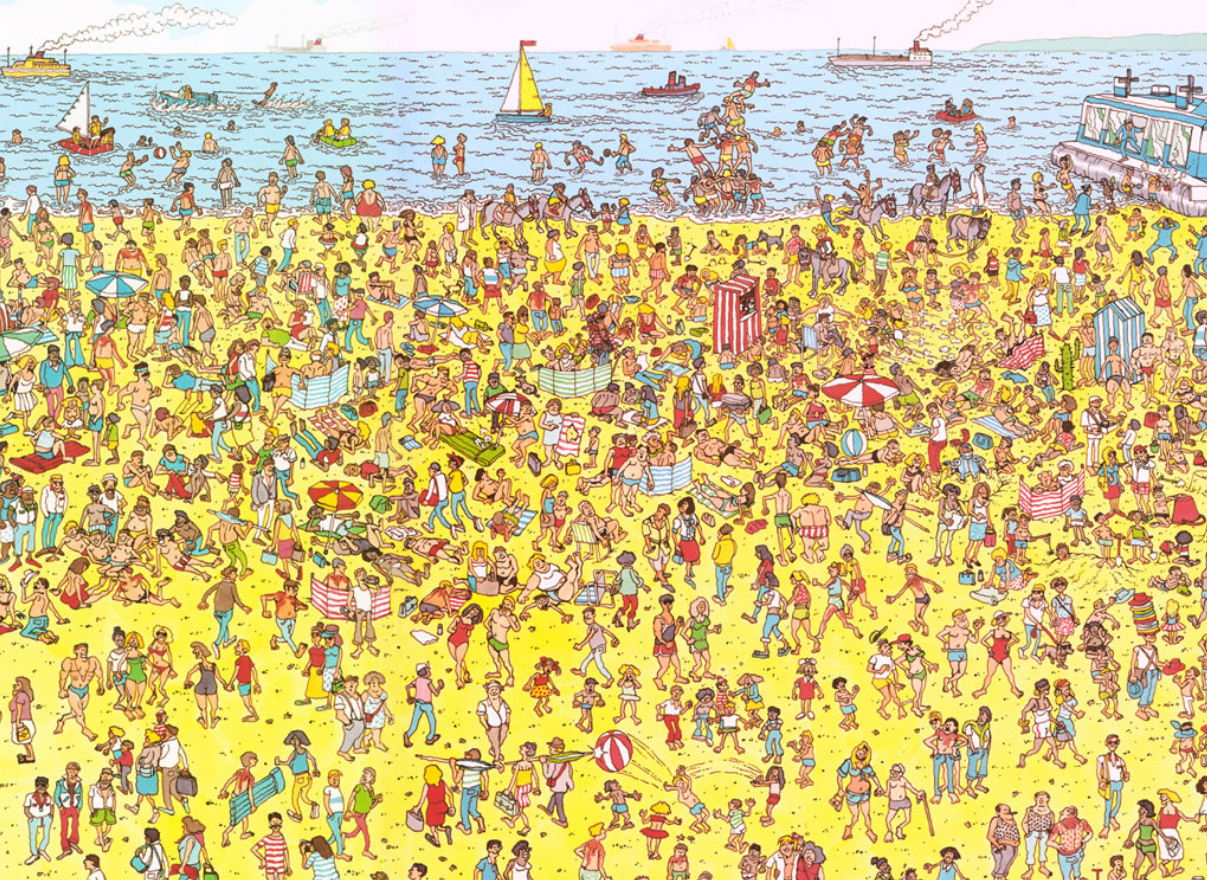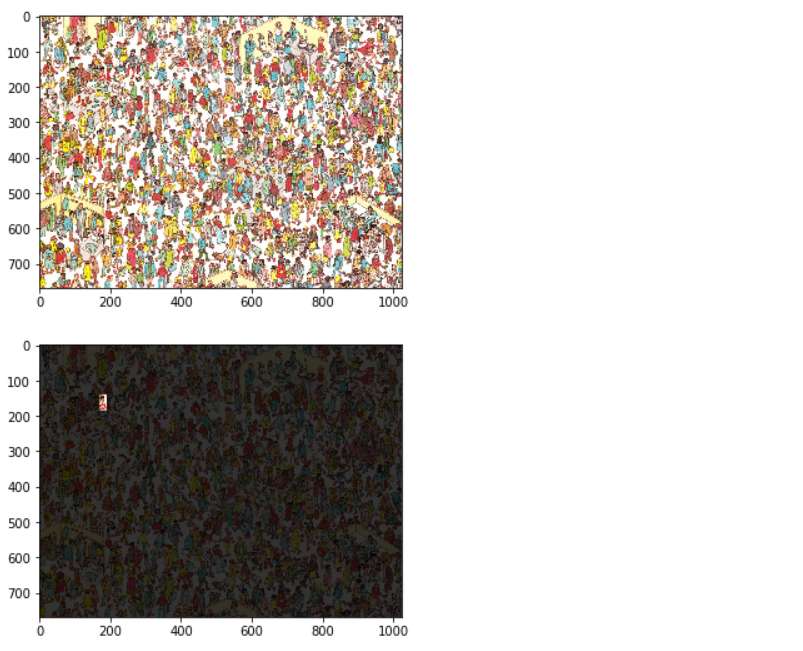# Where’s Wally Problem using Mahotas

In this article we will see how we can find the wally in the given image. Where’s Wally?, also called Where’s Waldo? in North America is a British puzzle books. The books consist of a series of detailed double-page spread illustrations showing dozens or more people doing a variety of amusing things at a given location. Readers are challenged to find a character named Wally hidden in the group.
Image used in the program –Wally Description : Wally is identified by his red-and-white-striped shirt, bobble hat, and glasses, but many illustrations contain red herrings involving deceptive use of red-and-white striped objects.
In order to do this we will use mahotas library. Mahotas is a computer vision and image processing library for Python. It includes many algorithms implemented in C++ for speed while operating in numpy arrays and with a very clean Python interface.
Command to install mahotas –

```pip install mahotas
```

Below is the implementation –

 `# importing required libraries ` `from` `pylab ``import` `imshow, show ` `import` `mahotas ` `import` `mahotas.demos ` `import` `numpy as np ` ` `  `# loading the image  ` `wally ``=` `mahotas.demos.load(``'wally'``) ` ` `  `# showing the original image ` `imshow(wally) ` `show() ` ` `  `# getting float type value  ` `# float values are better to use ` `wfloat ``=` `wally.astype(``float``) ` ` `  `# spiltting image into red, green and blue channel ` `r, g, b ``=` `wfloat.transpose((``2``, ``0``, ``1``)) ` ` `  `# white channel ` `w ``=` `wfloat.mean(``2``) ` ` `  `# pattern of wally shirt ` `# pattern + 1, +1, -1, -1 on vertical axis ` `pattern ``=` `np.ones((``24``, ``16``), ``float``) ` `for` `i ``in` `range``(``2``): ` `    ``pattern[i::``4``] ``=` `-``1` ` `  `# convolve with the red minus white ` `# increase the response where shirt is ` `v ``=` `mahotas.convolve(r``-``w, pattern) ` ` `  `# getting maximum value  ` `mask ``=` `(v ``=``=` `v.``max``()) ` ` `  `# creating mask to tone down the image  ` `# except the region where wally is ` `mask ``=` `mahotas.dilate(mask, np.ones((``48``, ``24``))) ` ` `  `# subtraction mask from the wally ` `np.subtract(wally, .``8` `*` `wally ``*` `~mask[:, :, ``None``],  ` `                   ``out ``=` `wally, casting ``=``'unsafe'``) ` ` `  `# show the new image ` `imshow(wally) ` `show() `

Output :My Personal Notes arrow_drop_upCheck out this Author's contributed articles.

If you like GeeksforGeeks and would like to contribute, you can also write an article using contribute.geeksforgeeks.org or mail your article to contribute@geeksforgeeks.org. See your article appearing on the GeeksforGeeks main page and help other Geeks.

Please Improve this article if you find anything incorrect by clicking on the "Improve Article" button below.

Improved By : drakeerv3

Article Tags :

1

Please write to us at contribute@geeksforgeeks.org to report any issue with the above content.